Astronomy 162: Introduction to Stars, Galaxies, & the Universe Prof. Richard Pogge, MTWThF 9:30

# Lecture 5: Distances of the Stars

## Key Ideas

Distance is the most important & most difficult quantity to measure in Astronomy

Method of Trigonometric Parallaxes
Direct geometric method of finding distances

Units of Cosmic Distance:
Light Year
Parsec (Parallax second)

## Why are Distances Important?

Distances are necessary for estimating:
• Total energy emitted by an object (Luminosity)
• Masses of objects from their orbital motions
• True motions through space of stars
• Physical sizes of objects
The problem is that distances are very hard to measure...

## The problem of measuring distances

Question:
How do you measure the distance of something that is beyond the reach of your measuring instruments?

Examples of such problems:

• Large-scale surveying & mapping problems.
• Military range finding to targets
• Measuring distances to any astronomical object

You resort to using GEOMETRY to find the distance.

## The Method of Trigonometric Parallaxes

Nearby stars appear to move with respect to more distant background stars due to the motion of the Earth around the Sun. This apparent motion (it is not "true" motion) is called Stellar Parallax.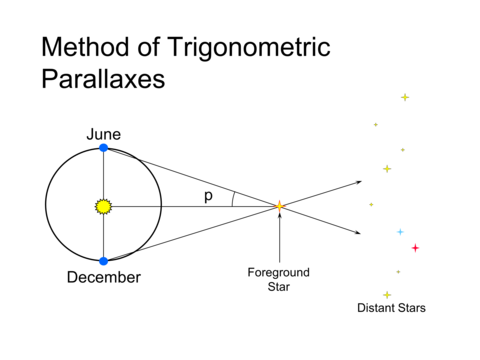(Click on the image to view at full scale [Size: 177Kb])

In the picture above, the line of sight to the star in December is different than that in June, when the Earth is on the other side of its orbit. As seen from the Earth, the nearby star appears to sweep through the angle shown. Half of this angle, is the parallax, p.

## Parallax decreases with Distance

As the distance to a star increases, the its parallax decreases. This is easy to see in the following two figures:(Click on the image to view at full scale [Size: 165Kb])

In the upper figure, the star is about 2.5 times nearer than the star in the lower figure, and has a parallax angle which is 2.5 times larger.

This movie demonstrates parallaxes graphically (beware: it is big, don't try to download it over a slow network connection).

This gives us a means to measure distances directly by measuring the parallaxes of nearby stars.

We call this powerful direct distance technique the Method of Trigonometric Parallaxes.

## Stellar Parallaxes

Because the even the nearest stars are very far away, the largest measured parallaxes is very small; less than an arcsecond.

For example, the nearest star, Proxima Centauri, has a parallax of 0.772-arcsec (the largest parallax observed for any star).

First parallax observed 1837 (Friedrich Bessel) for the star 61 Cygni.

We use photography and digital imaging techniques to measure parallaxes today. Increasingly, we measure parallaxes from space to avoid blurring due to the Earth's atmosphere.

## Parallax Formula:

We saw before that the smaller the parallax, the larger the distance. We can express this as a simple formula: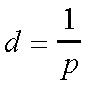Where:

p = parallax angle in arcseconds

d = distance in "Parsecs"

Writing our parallax formula in this way allows us to define a new "natural" unit for distances in astronomy: the Parallax-Second or Parsec.

## Parallax Second = Parsec (pc)

Fundamental unit of distance in Astronomy
"A star with a parallax of 1 arcsecond has a distance of 1 Parsec."

1 parsec (pc) is equivalent to:

206,265 AU
3.26 Light Years
3.086x1013 km

## Light Years

An alternative unit of astronomical distance is the Light Year (ly).
"1 Light Year is the distance traveled by light in one year."

1 light year (ly) is equivalent to:

0.31 pc
63,270 AU

The light year is used primarily by writers of popular science books and science fiction writers. It is rarely used in research astronomy.

The reason is that the parsec is directly derived from the quantity that is being measured (the stellar parallax angle), whereas the light-year must be derived from having previously measured the distance in parsecs. In this way, the parsec is a more "natural" unit to use than the light year.

## Examples of Parallax Distances:

1. The star alpha Centauri has a parallax of p=0.742-arcsec: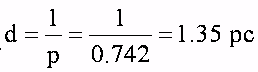2. A star is measured to have a parallax of p=0.02-arcsec: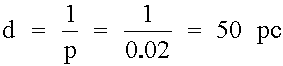## Limitations

If the stars are too far away, the parallax can be too small to measure accurately.

In general, the greater the distance, the smaller the parallax, and so the less precise the distance measurement will be.

The smallest parallax measurable from the ground is about 0.01-arcsec. This means that from the ground, the method of Trigonometric Parallaxes has the following limitations.

• good out to 100 pc
• only get 10% distances out to a few parsecs.
• only a few hundred stars are this close

## Hipparcos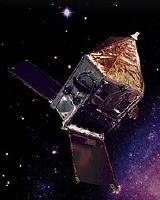The Hipparcos satellite (launched by the European Space Agency in 1989) measured precision parallaxes to an accuracy of about 0.001-arcsec.

• Hipparcos measured parallaxes for about 100,000 stars
• Got 10% accuracy distances out to about 100 pc
• Good distances for bright stars out to 1000 pc.

Hipparcos represented a great leap in our knowledge of the distances (and motions) of nearby stars. The catalog was just released in late 1997, and is already having an impact on many areas of astronomy that rely in accurate distances.

## The Future

Gaia

Gaia is a European follow-up mission to Hipparcos that was launched on 19 December 2013, reaching its orbital station at the Earth/Sun L2 point in January 2014, and began science operations in July 2014. Its goal is to conduct what amounts to a Galactic census. Gaia is undertaking an all-sky survey of the Galaxy that build up high-precision angular measurements through repeated measurements. The final Gaia data catalog will not be completed until about 2020.

Mission Goals:

• Measure positions and motions for about 1 Billion Stars
• Measure parallaxes for >200 Million Stars
• Max precision of 10 microarcseconds
• This means 10% or better distances out to 10,000pc